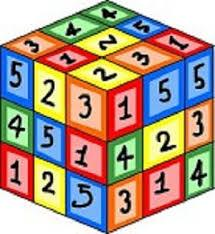# Methodius 3758

Milan and Methodius are now 70 years old. Seven years ago, Milan was 1.8 times older than Methodius. Determine how old Milan is now and how many Metoděj is.

a =  43
b =  27

### Step-by-step explanation:

a+b=70
a-7 = 1.8 ·(b-7)

a+b = 70
a-1.8b = -5.6

Row 2 - Row 1 → Row 2
a+b = 70
-2.8b = -75.6

b = -75.6/-2.8 = 27
a = 70-b = 70-27 = 43

a = 43
b = 27

Our linear equations calculator calculates it.Did you find an error or inaccuracy? Feel free to write us. Thank you!

Tips for related online calculators
Do you have a system of equations and looking for calculator system of linear equations?
Do you want to convert time units like minutes to seconds?